# Boks integral

(diff) ← Older revision | Latest revision (diff) | Newer revision → (diff)
One of the generalizations of the Lebesgue integral, proposed by A. Denjoy (1919) and studied in detail by T.J. Boks (1921). A real-valued functionon a segmentis periodically extended (with period length) to the entire straight line. For an arbitrary subdivisionof, for an arbitrary selection of points,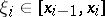, and an arbitrary, the following sum is constructed: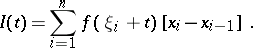If, for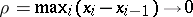,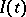converges in measure to a definite limit, the numberis said to be the Boks integral (-integral) ofover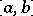. Thus, the Boks integral is an integral of Riemann type and is a generalization of the Riemann integral.
The Boks integral represents a considerable extension of the Lebesgue integral: Any summable function is-integrable and these integrals coincide, but there exist non-summable-integrable functions; in particular, if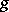is the function conjugate with a summable function, then it is-integrable and the coefficients of the series conjugate with the Fourier series ofare the coefficients of the respective Fourier series (in the sense of-integration) of(A.N. Kolmogorov). The theory of the Boks integral was not further developed, since the-integral proved to be more convenient for the integration of functions conjugate with summable functions.